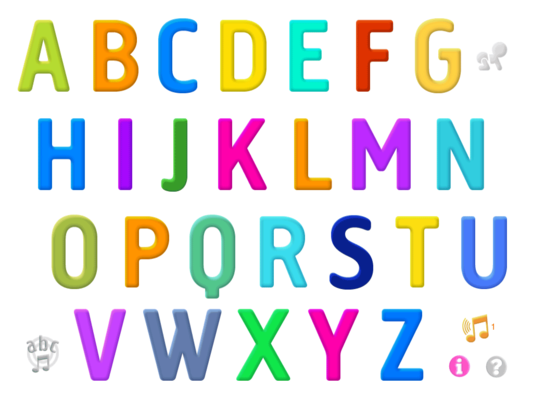ABC's
4 years ago
Save
Edit
Host a game
Live GameLive
Homework
Solo Practice
Practice12 QuestionsShow answers
• Question 1
60 seconds
Q. L, M, N, O, P, __, __, __. What are the next 3 letters?
T, U, V
Q,R,S
C,D,E
W,X,Y
• Question 2
60 seconds
Q. D,E,F,G,__,__,__. What is the next 3 letters?
H,I,J
H,L,M
I,P,O
A,B,C
• Question 3
60 seconds
Q. A,B,C,__,__, F,G. What letters are missing?
H,I
J,K
L,M
D,E
• Question 4
60 seconds
Q. H,I,J,__,__,M,N. What are the missing letters?
K,L
G,K
K,P
L,O
• Question 5
60 seconds
Q. T,U,V,W,__,__,__. What are the next 3 letters?
S,Y,Z
X,Y,Z
Y,Z,S
Z,S,R
• Question 6
60 secondsQ.
t
p
g
• Question 7
60 secondsQ.
d
c
h
• Question 8
60 secondsQ.
r
b
h
• Question 9
60 secondsQ.
c
s
z
• Question 10
60 secondsQ.
j
l
g
• Question 11
60 secondsQ.
t
r
k
• Question 12
60 secondsQ.